Courses

# Test: ILD & Rolling Loads -1

## 10 Questions MCQ Test Topicwise Question Bank for Civil Engineering | Test: ILD & Rolling Loads -1

Description
This mock test of Test: ILD & Rolling Loads -1 for GATE helps you for every GATE entrance exam. This contains 10 Multiple Choice Questions for GATE Test: ILD & Rolling Loads -1 (mcq) to study with solutions a complete question bank. The solved questions answers in this Test: ILD & Rolling Loads -1 quiz give you a good mix of easy questions and tough questions. GATE students definitely take this Test: ILD & Rolling Loads -1 exercise for a better result in the exam. You can find other Test: ILD & Rolling Loads -1 extra questions, long questions & short questions for GATE on EduRev as well by searching above.
QUESTION: 1

### When a series of wheel loads crosses a simply supported girder, the maximum bending moment under any given wheel load occurs when

Solution:

For a simply supported girder, the absolute maximum bending moment for a series of wheel loads occurs at a section at which the centre of gravity of the load system is midway between the centre of span and wheel load under consideration. At that section the considered wheel load and as a reference to the load other loads are placed in the influence line diagram to calculate the absolute maximum bending moment.

QUESTION: 2

Solution:
QUESTION: 3

### The ordinates of influence line diagram for bending moment always have the dimension of

Solution:
QUESTION: 4

In a 3 hinged arch
1. Influence line for horizontal thrust is parabolic.
2. Maximum horizontal thrust occurs when the unit load is at crown.
3. Maximum sagging moment occurs when the unit load is at section itself.

Which of these statements is correct?

Solution:
QUESTION: 5

The influence line diagram for bending moment at section X(Mx), at a distance of 4 m from the left support of a simply supported girder AB is shown in figure below. A uniformly distributed load of intensity 2t/m longer than the span crosses the girder from left to right.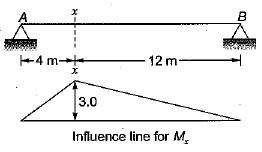The maximum bending moment at section X is equal to

Solution:

The maximum bending moment at section-Xwill occur when UDL occupies the whole span.
Maximum bending = Area under the influence line diagram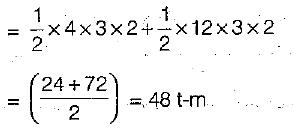QUESTION: 6

The Muller-Breslau principle can be used to
1. Determine the shape of the influence line
2. Indicate the parts of the structure to be loaded to obtain the maximum effect
3. Calculate the ordinates of the influence lines

Which of the these statements is/are correct?

Solution:
QUESTION: 7

The influence line for shear at section x (Fx) at a distance of 4 m from the left supported girder AB is shown in figure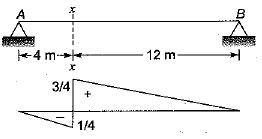The shear force at section oe due to a uniformly distributed dead load of intensity 2 t/m covering the entire span will be

Solution:

SF will be equal to-the area under the influence line diagram,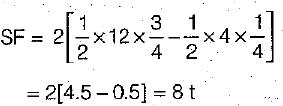QUESTION: 8

A beam with a cantilevered arm BC supporting a freely supported end span CD is shown in the figure. Which one of the figure represents the influence line diagram for shear force at A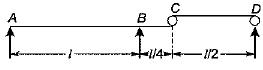Solution:
QUESTION: 9

Which one of the following represents the correct influence line for bending moment at point C for the beam shown in the figure below: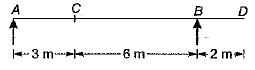Solution:
QUESTION: 10

Three wheel loads 10t, 26t and 24t spaced 2m apart roll on a girder from left to right with the 10t load leading. The girder has a span of 20 meter. For the condition of maximum bending moment at a section 8 meter from the left end.

Solution:

Maximum bending moment at a section occurs when a particular load is on the section which changes the ratio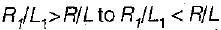as the load passes over the section .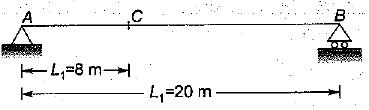where R1, → resultant of load on left sid e of section Resultant of all loads (R)
= 10 + 26 + 24 = 60t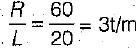When 10t load crosses section C.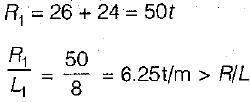When 26t load crosses the section C,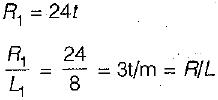It means that maximum bending moment is obtained when 26t load is on the section.